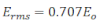# Root Mean Square Value Of AC (rms value)

Since the average value of AC over a cycle is zero. For this reason, a more appropriate quantity is to be defined called Root mean square value of AC.
The root mean square value of AC is defined in terms of DC that does the same work at the same interval of time as it would be done by AC at the same time.
Thus the rms value of AC is equivalent to that steady current which when passed through a resistor would produce the same heat that would be done by the AC in passing through the same resistor in similar condition.

To calculate the mean value of ac, Let us consider an ac given by: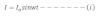the small amount of work done by an ac in small time dt is: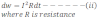So total work done is obtained by integrating equation ii from t = 0 to t = T we get,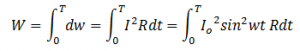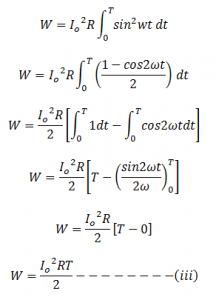If Irms be the RMS value of ac then it would do the same work as in equation iii while passing through R in time T,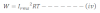Now from equation iii and iv we get,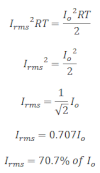This relation shows that the root mean square value of ac is about 70.7% of its pick value.

Similarly the root mean square value of alternating emf is: